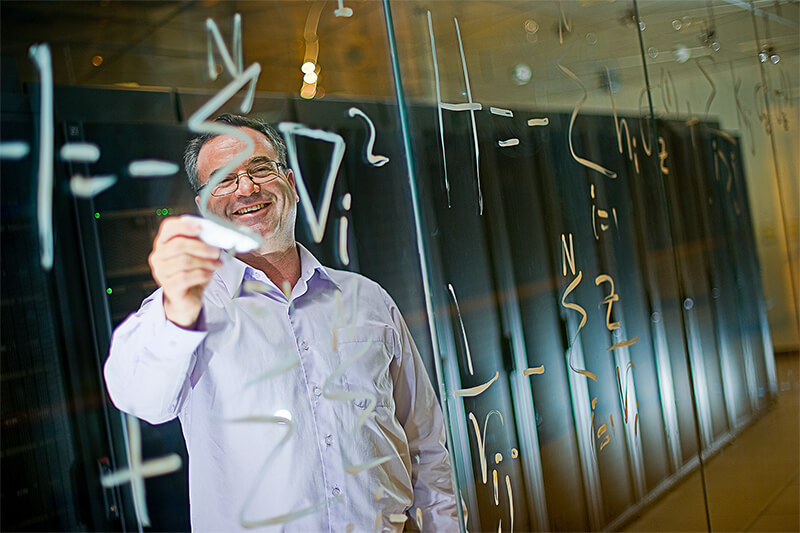Posted in | Quantum Computing

# New Theory Could Enable More Systematic Design of Quantum Algorithms

Back in 2019, Google stated that it was the first to showcase a quantum computer carrying out a calculation surpassing the capabilities of the most powerful existing supercomputers.Sabre Kais’ research group at Purdue is developing quantum algorithms and quantum machine learning methods. Image Credit: Purdue University.

Researchers at Purdue University say that in most cases, developing a quantum algorithm that is capable of surpassing a classical computer is a coincidental process. To give more direction to this process and reduce the arbitrariness, the Purdue team put together a new theory that may ultimately pave the way toward a more methodical design of quantum algorithms.

Published in the Advanced Quantum Technologies journal, the new theory is the first recognized attempt to establish which quantum states can be developed and processed with a satisfactory number of quantum gates to beat a classical algorithm.

Physicists call this concept of having an accurate number of gates to regulate each state as “complexity.” The complexity of a quantum algorithm is largely dependent on the complexity of quantum states involved in the algorithm; therefore, the theory could thus bestow order to the search for quantum algorithms by defining which quantum states match those complexity criteria.

An algorithm is a string of steps to carry out a calculation. The algorithm is typically executed on a circuit.

Circuits in classical computers include gates that swap bits to either a 0 or 1 state. On the other hand, a quantum computer depends on computational units known as “qubits” that store 0 and 1 states concurrently in superposition, enabling more data to be processed.

A quantum computer is judged as faster than a classical computer based on its simpler data processing ability, characterized by the huge decrease in the number of quantum gates in a quantum circuit than in a classical circuit.

The number of gates in circuits in classical computers grows exponentially in relation to the size of the problem of interest. This exponential model increases so amazingly fast that it becomes physically difficult to manage for even a reasonably sized problem of interest.

For example, even a small protein molecule may contain hundreds of electrons. If each electron can only take two forms, then to simulate 300 electrons would require 2300 classical states, which is more than the number of all the atoms in the universe.

Sabre Kais, Professor in Chemistry and Member of Purdue Quantum Science and Engineering Institute, Purdue University

When it comes to quantum computers, there is a technique for quantum gates to increase “polynomially”—instead of exponentially like a classical computer—with the size of the problem (like the number of electrons in the previous example). “Polynomial” means that there would be radically fewer steps (gates) necessary to process the same amount of data, making a quantum algorithm better than a classical algorithm.

Thus far, scientists have not had a proper way to detect which quantum states could fulfill this requirement of polynomial complexity.

There is a very large search space for finding the states and sequence of gates that match up in complexity to create a useful quantum algorithm capable of performing calculations faster than a classical algorithm.

Sabre Kais, Professor in Chemistry and Member of Purdue Quantum Science and Engineering Institute, Purdue University

The research team of Kais is creating quantum algorithms and quantum machine learning approaches.

Kais and Zixuan Hu, a Purdue postdoctoral associate, applied the new theory to detect a large group of quantum states that have polynomial complexity. Moreover, they demonstrated that these states are likely to share a coefficient feature that could be applied to properly identify them while developing a quantum algorithm.

Given any quantum state, we are now able to design an efficient coefficient sampling procedure to determine if it belongs to the class or not.

Zixuan Hu, Postdoctoral Associate, Purdue University

This research received financial support from the U.S. Department of Energy (Office of Basic Energy Sciences) under award no. DE-SC0019215. The Purdue Quantum Science and Engineering Institute is part of Purdue’s Discovery Park.

## Journal Reference:

Hu, Z., et al. (2020) Characterization of quantum states based on creation complexity. Advanced Quantum Technologies. doi.org/10.1002/qute.202000043.

Source: https://www.purdue.edu

## Tell Us What You Think

Do you have a review, update or anything you would like to add to this news story?